# Preface

This section provides some analysis of spring-mass systems under quadratic restoring force.

Introduction to Linear Algebra with Mathematica

When modeling a spring-mass system, we usually need to take into account resistance forces acting on a spring and a mass (friction). These forces are typically noninear and their modeling requires variable approximations at different stages. Previously, we discussed viscous damping and cubic restoring force in the Duffing model. In this section, we consider the spring's restoring force that is only mildly nonlinear. This leads to an even function (quadratic) as a restoring force and corresponding differential equation
\begin{equation} \label{EqQuadratic.1} \ddot{x} + k\,\dot{x} + x + \varepsilon\,x^2 = 0, \end{equation}
where the term $$k\,\dot{x}$$ models viscous damping. Here the mass of the weight has been normalized to be 1 and without any loss of generality the coefficient of x has been assumed to be 1 (it is at most a rescaling of the time variable to do so). In this section, we consider different quadratic models for damping forses that arise in spring-mass systems. First, we start with some examples.
$\ddot{x} + x -3\,x^2 = 0 . \tag{1.1}$
Upon introducing an auxiliary dependent avriable (velocity), we reduce the given differential equation to a system of first order equations:
$\begin{split} \dot{x} &= v , \\ \dot{v} &= -x + 3\,x^2 . \end{split}$
This system has two critical points
$O(0,0) \qquad \mbox{and} \qquad P(1/3, 0) .$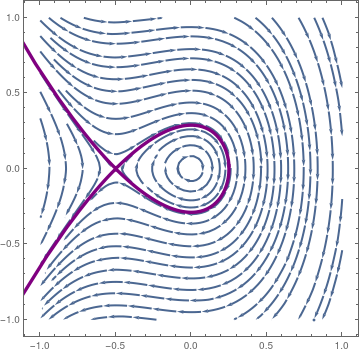As the phase portrate shows, the origin is a center, but P is a saddle point. We plot the phase portrate for the equation $\ddot{x} + x +3\,x^2 = 0 . \tag{1.2}$ along with the separatrix: sp = StreamPlot[{v, -x - 2*x^2}, {x, -1, 1}, {v, -1, 1}, StreamStyle -> Thick]; s1 = NDSolve[{x''[t] + x[t] + 2*x[t]^2 == 0, x == 0.2495, x' == 0.0198}, x, {t, -14, 14}]; pp1 = ParametricPlot[Evaluate[{x[t], x'[t]} /. s1], {t, 0, 13}, PlotRange -> {{-1, 1}, {-1, 1}}, PlotStyle -> {Thickness[0.01], Purple}]; mm1 = ParametricPlot[Evaluate[{x[t], x'[t]} /. s1], {t, 0, -13}, PlotRange -> {{-1, 1}, {-1, 1}}, PlotStyle -> {Thickness[0.01], Purple}]; Show[sp, pp1, mm1]We plot the phase portrate for the equation $\ddot{x} + x -3\,x^2 = 0 . \tag{1.2}$ along with the separatrix: s3 = NDSolve[{x''[t] + x[t] - 3*x[t]^2 == 0, x == -0.167, x' == 0}, x, {t, -14, 14}]; vp = VectorPlot[{v, -x + 3*x^2}, {x, -1, 1}, {v, -1, 1}, PlotTheme -> "Web", RegionFillingStyle -> Gray, VectorPoints -> Fine]; pp3 = ParametricPlot[Evaluate[{x[t], x'[t]} /. s3], {t, 0, 13}, PlotRange -> {{-1, 1}, {-1, 1}}, PlotStyle -> {Thickness[0.01], Blue}]; mm3 = ParametricPlot[Evaluate[{x[t], x'[t]} /. s3], {t, 0, -13}, PlotRange -> {{-1, 1}, {-1, 1}}, PlotStyle -> {Thickness[0.01], Blue}]; Show[vp, pp3, mm3]

■
Example 2: Now we consider the non-linear differential equation with viscous damping
$\ddot{x} + x + \frac{1}{3}\,\dot{x} -3\,x^2 = 0 . \tag{1.2}$
We plot its phase portrait and similar plot for
$\ddot{x} + x + \frac{1}{3}\,\dot{x} +3\,x^2 = 0 .$
sp = StreamPlot[{v, -x - v/3 + 3*x^2}, {x, -0.5, 0.5}, {v, -0.5, 0.5}, StreamStyle -> Thick] VectorPlot[{v, -x - v/3 - 3*x^2}, {x, -1, 1}, {v, -1, 1}, PlotTheme -> "Web", RegionFillingStyle -> Gray, VectorPoints -> Fine];
s1 = NDSolve[{x''[t] + x[t] + x'[t]/3 - 3*x[t]^2 == 0, x == 0.4, x' == -0.0609}, x, {t, -10, 10}];
s2 = NDSolve[{x''[t] + x[t] + x'[t]/3 - 3*x[t]^2 == 0, x == 0.25, x' == -0.463}, x, {t, -10, 10}];
pp1 = ParametricPlot[Evaluate[{x[t], x'[t]} /. s1], {t, 0, 10}, PlotRange -> {{-1, 1}, {-1, 1}}, PlotStyle -> {Thickness[0.01], Purple}];
pp2 = ParametricPlot[Evaluate[{x[t], x'[t]} /. s2], {t, 0, 7.0}, PlotRange -> {{-1, 1}, {-1, 1}}, PlotStyle -> {Thickness[0.01], Purple}];
mm1 = ParametricPlot[Evaluate[{x[t], x'[t]} /. s1], {t, 0, -10}, PlotRange -> {{-1, 1}, {-1, 1}}, PlotStyle -> {Thickness[0.01], Purple}];
Show[sp, pp1, mm1, pp2]
sp = StreamPlot[{v, -x - v/3 - 3*x^2}, {x, -0.5, 0.5}, {v, -0.5, 0.5}, StreamStyle -> Thick] VectorPlot[{v, -x - v/3 - 3*x^2}, {x, -1, 1}, {v, -1, 1}, PlotTheme -> "Web", RegionFillingStyle -> Gray, VectorPoints -> Fine];
s1 = NDSolve[{x''[t] + x[t] + x'[t]/3 + 3*x[t]^2 == 0, x == -0.4, x' == -0.0609}, x, {t, -10, 10}];
s2 = NDSolve[{x''[t] + x[t] + x'[t]/3 + 3*x[t]^2 == 0, x == -0.25, x' == 0.463}, x, {t, -10, 10}];
pp1 = ParametricPlot[Evaluate[{x[t], x'[t]} /. s1], {t, 0, 10}, PlotRange -> {{-1, 1}, {-1, 1}}, PlotStyle -> {Thickness[0.01], Purple}];
pp2 = ParametricPlot[Evaluate[{x[t], x'[t]} /. s2], {t, 0, 7.0}, PlotRange -> {{-1, 1}, {-1, 1}}, PlotStyle -> {Thickness[0.01], Purple}];
mm1 = ParametricPlot[Evaluate[{x[t], x'[t]} /. s1], {t, 0, -10}, PlotRange -> {{-1, 1}, {-1, 1}}, PlotStyle -> {Thickness[0.01], Purple}];
Show[sp, pp1, mm1, pp2]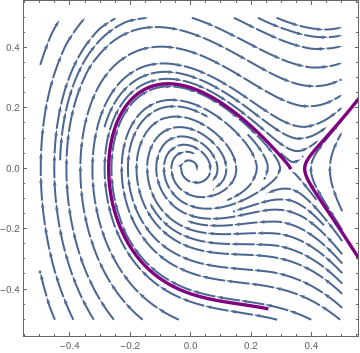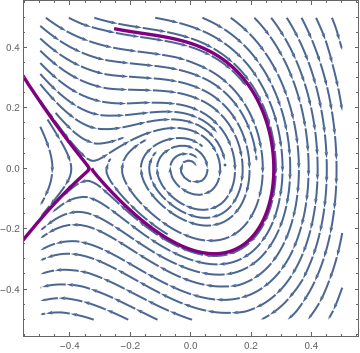Phase portrait for $$\ddot{x} + x + \frac{1}{3}\,\dot{x} -3\,x^2 = 0 ,$$ wityh separatrix. Phase portrait for $$\ddot{x} + x + \frac{1}{3}\,\dot{x} +3\,x^2 = 0 ,$$ with separatrix.

■

To analyze Eq.\eqref{EqQuadratic.1}, we convert it to the system of first order ODEs:

\begin{equation} \label{EqQuadratic.2} \begin{split} \dot{x} &= y , \\ \dot{y} &= -k\,y -x - \varepsilon\,x^2 . \end{split} \end{equation}
System \eqref{EqQuadratic.2} has two critical points
$O \left( 0,0 \right) \qquad \mbox{and} \qquad S \left( - \frac{1}{\varepsilon} , 0 \right) \$
Since the Jacobian for system \eqref{EqQuadratic.2} is
${\bf J}(x,y) = \begin{bmatrix} 0& \phantom{-}1 \\ -1 -2\varepsilon x & -k \end{bmatrix} \qquad \Longrightarrow \qquad {\bf J}(0,0) = \begin{bmatrix} 0&\phantom{-}1 \\ -1 & -k \end{bmatrix} \quad {\bf J}(-1/\varepsilon ,0) = \begin{bmatrix} 0&\phantom{-}1 \\ 1& -k \end{bmatrix}$
The eigenvalues of matrix J(0, 0) are $$\displaystyle \lambda = \frac{1}{2} \left( -k \pm \sqrt{k^2 - 4} \right) .$$
Eigenvalues[{{0, 1}, {-1, -k}}]
{1/2 ( -k - Sqrt[-4 + k^2]), 1/2 (k + Sqrt[-4 + k^2])}
When k is positive, the origin becomes asymptotically stable spiral equilibrium. However, when k = 0, it is a center (stable).

The eigenvalues of matrix J(−1/ε, 0) are $$\displaystyle \lambda = \frac{1}{2} \left( -k \pm \sqrt{k^2 + 4} \right) .$$ Therefore, it is a saddle point because eigenvalues are real and of different sign.

Eigenvalues[{{0, 1}, {1, -k}}]
{1/2 (-k - Sqrt[4 + k^2]), 1/2 (-k + Sqrt[4 + k^2])}

Viscous damping is a mathematical convenience and its use results from its simplicity. However, it is not completely accurate, especially for velocities that are large. For velocities that are larger, the frictional force is probably proportional to the square of the velocity, as opposed to a linear relationship like in viscous damping. For larger velocities, quadratic damping is used. Quadratic damping is more realistic and applicable to many models, including motion through fluids and gases. For example modelling the drag on a bird flying through strong winds. For quadratic damping, because it always opposes the direction of the motion, there is a jump discontinuity when velocity equals zero.

To calculate the maximum amplitudes of the oscillations of quadratically damped harmonic oscillators and quadratically damped pendulums, an approach with energy is used. To start, the jump discontinuity is ignored and we find a generalized energy function for

$\ddot{x} + p(x)\,\dot{x} + f(x) = 0$
using an integrating factor and integration by parts. For quadratic damping, we must take into account the frictional force always opposing the direction of motion, we use signum function. So
$\ddot{x} + c\,x\left\vert \dot{x} \right\vert + f(x) = 0$
With this function, the critical values do not depend on the coefficient of friction, which makes it very generalizable for different applications. This approach is used for the harmonic oscillator and pendulum to find the maximum amplitude of oscillations.

Inhomogeneous equation

The model of mechanical or electronic oscillations, typically that of a suspended spring with weight attached, is commonly presented in beginning courses on differential equations and the linear case is completely understood. Here one introduces Hooke’s Law and Newton’s Law to obtain the standard linear spring model and one introduces the notions of periodic response, beats and resonance, and when viscous damping and forcing are added, critical damping and steady states. There is a new emphasis in such courses on systems and nonlinear models due to the wide availability of fast hardware and powerful software, and thus nonlinear spring models are natural to introduce in an attempt to make the spring model more physically meaningful while keeping things reasonably simple. The nonlinear case is more interesting as the range of resultant motions is much wider. We start with a simpel case, ignore any damping and concentrate upon the equation

\begin{equation} \label{EqQuadratic.3} \ddot{x} + x + \varepsilon x^2 = \Gamma\, \sin \left( \omega t \right) \qquad \mbox{or} \qquad \ddot{x} + x + \varepsilon x^2 = \Gamma\, \cos \left( \omega t \right) . \end{equation}

To get an idea of what responses are available, we begin with some examples that have interesting trajectories in the phase plane. The first example is that with a harmonic solution having a typical harmonic trajectory.

$\ddot{x} + x - \frac{1}{6}\, x^2 = \frac{1}{2}\,\sin \left( \frac{t}{5} \right) , \qquad x(0) = a, \quad \dot{x}(0) = 0 . \tag{3.1}$
■
$\ddot{x} + 2\,x -\frac{3}{2}\,x^2 = \frac{1}{2}\,\sin \left( \frac{t}{5} \right) . \tag{4.1}$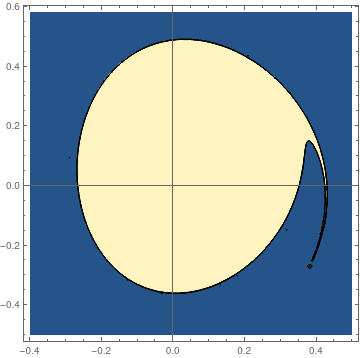pfun = ParametricNDSolveValue[{x''[t] + 2 x[t] - (3*x[t])^2/6 == (1/2)*Sin[t/5], x == a, x' == b}, x, {t, 0, 100}, {a, b}]; fun[a_?NumericQ, b_?NumericQ] := Module[{res}, res = Quiet[pfun[a, b]]; Boole[res["Domain"] === {{0., 100.}}]]; plot = ContourPlot[fun[a, b], {a, -0.4, 0.5}, {b, -0.5, 0.58}, PlotPoints -> 50, MaxRecursion -> 3, Axes -> True, AxesOrigin -> {0, 0}] Domain of stability. Mathematica code.

Now we consider slightly different equation
$\ddot{x} + x -\frac{1}{3}\,x^2 = \frac{1}{9}\,\sin \left( \frac{t}{8} \right) . \tag{4.2}$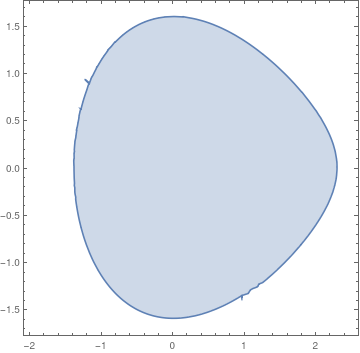pfun = ParametricNDSolveValue[{3 x''[t] + 3 x[t] - (x[t])^2 == (1/3)* Sin[t/8], x == a, x' == b}, x, {t, 0, 100}, {a, b}]; fun[a_?NumericQ, b_?NumericQ] := Module[ {res}, (* determine if domain is valid or invalid and return 1 or 0 respectively *) res = Quiet[pfun[a, b]]; Boole[res["Domain"] === {{0., 100.}}] ]; regionplot = RegionPlot[fun[a, b] >= 1, {a, -2.0, 2.5}, {b, -1.7, 1.7}] Domain of stability. Mathematica code.

For another input sine function, we obtain almost the same stability domain. However, for the following equation
$\ddot{x} + x -\frac{1}{3}\,x^2 = \frac{1}{9}\,\cos \left( \frac{t}{8} \right) + \frac{1}{9}\,\sin \left( \frac{t}{8} \right) , \tag{4.3}$
we get different shape.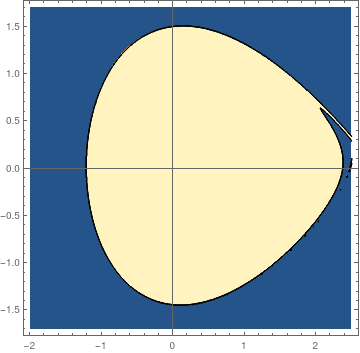pfun = ParametricNDSolveValue[{3 x''[t] + 3 x[t] - (x[t])^2 == (1/3)*Cos[t/8] + (1/3)*Sin[t/8], x == a, x' == b}, x, {t, 0, 100}, {a, b}]; fun[a_?NumericQ, b_?NumericQ] := Module[{res}, res = Quiet[pfun[a, b]]; Boole[res["Domain"] === {{0., 100.}}]]; plot = ContourPlot[fun[a, b], {a, -2.0, 2.5}, {b, -1.7, 1.7}, PlotPoints -> 50, MaxRecursion -> 3, Axes -> True, AxesOrigin -> {0, 0}] Domain of stability. Mathematica code.

We plot some typical trajectories.
sol2 = NDSolve[{3 x''[t] + 3 x[t] - (x[t])^2 == (1/3)*Sin[t/8], x == 0.2, x' == 0.0}, x, {t, 0, 30}];
ParametricPlot[Evaluate[{x[t], x'[t]} /. sol2], {t, 0, 30}, PlotStyle -> Thick]
sol2 = NDSolve[{3 x''[t] + 3 x[t] - (x[t])^2 == (1/3)*Sin[t/8], x == 2.293701, x' == 0}, x, {t, 0, 30}]
ParametricPlot[Evaluate[{x[t], x'[t]} /. sol2], {t, 0, 30}, PlotStyle -> Thick]
and finally, trajectory that starts outside the stability region:
sol2 = NDSolve[{3 x''[t] + 3 x[t] - (x[t])^2 == (1/3)*Sin[t/8], x == 2.31, x' == 0}, x, {t, 0, 30}];
ParametricPlot[Evaluate[{x[t], x'[t]} /. sol2], {t, 0, 13}, PlotTheme -> "Business"]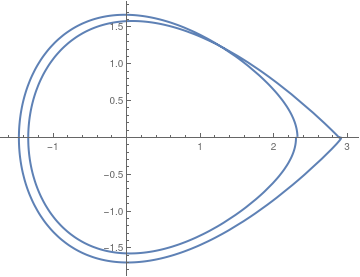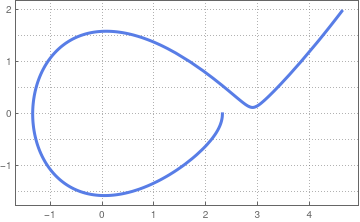We use manipulate command to determine the boundary of stability domain for another equation:
$\ddot{x} + \frac{3}{2}\,x -\frac{5}{2}\,x^2 = \frac{1}{5}\,\sin \left( \frac{5\,t}{2} \right) . \tag{4.4}$
pfun = ParametricNDSolveValue[{2*x''[t] + 3 x[t] - 5*(x[t])^2 == (2/5)*Sin[5*t/2], x == a, x' == b}, x, {t, 0, 100}, {a, b}];
fun[a_?NumericQ, b_?NumericQ] := Module[ {res}, (* determine if domain is valid or invalid and return 1 or 0 respectively *) res = Quiet[pfun[a, b]]; Boole[res["Domain"] === {{0., 100.}}] ];
regionplot = RegionPlot[fun[a, b] >= 1, {a, -0.3, 0.45}, {b, -0.55, 0.5}];
mesh = BoundaryDiscretizeGraphics[regionplot]
Manipulate[ ListPlot[coord[[1 ;; n]], PlotRange -> {{-0.3, 0.45}, {-0.55, 0.5}}], {{n, 50}, 1, Length[coord], 1}]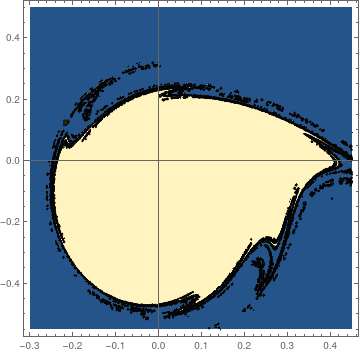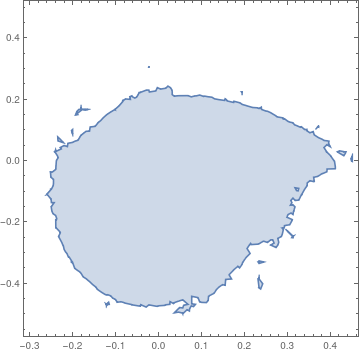On the other hand, the stability domain for the differential equation with cosine driving function is
$\ddot{x} + \frac{3}{2}\,x -\frac{5}{2}\,x^2 = \frac{1}{5}\,\cos \left( \frac{5\,t}{2} \right) . \tag{4.5}$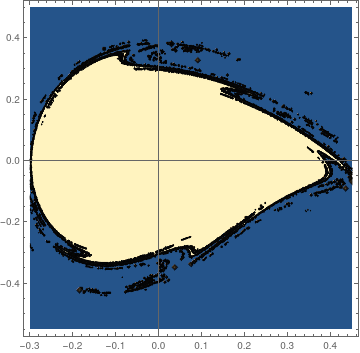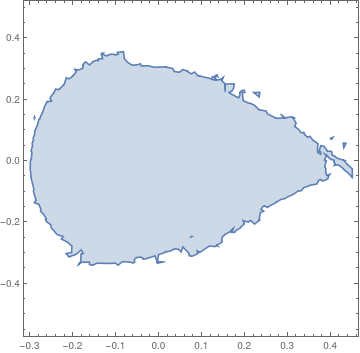■

Nonlinearity is typically introduced by assuming that the spring’s restoring force is nonlinear and perhaps the most common form is that of the Duffing spring equation

$\ddot{x} + c\,x\left\vert \dot{x} \right\vert + x + \epsilon x^3 = \gamma\,\sin \omega t .$
Example 5:    ■